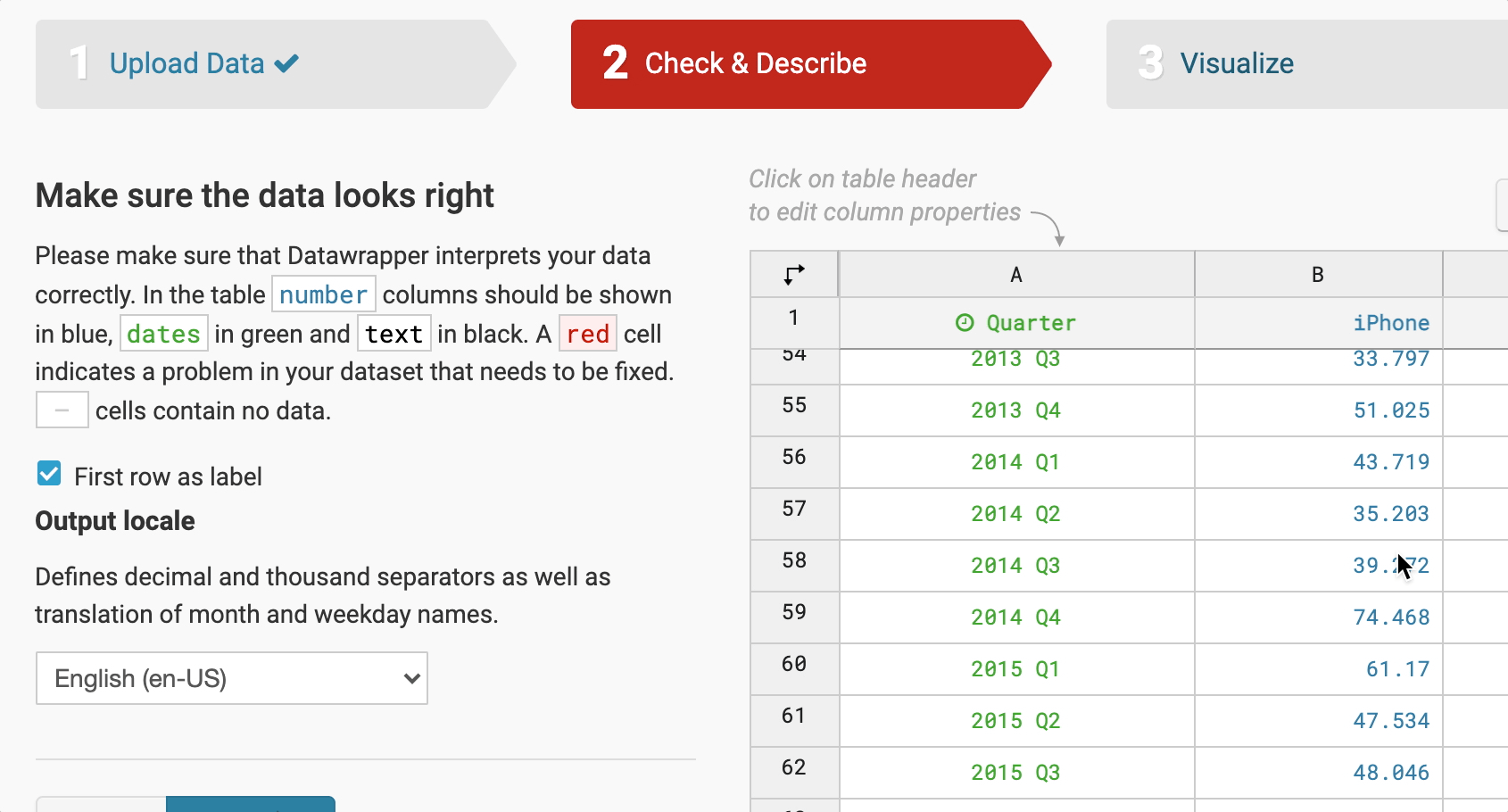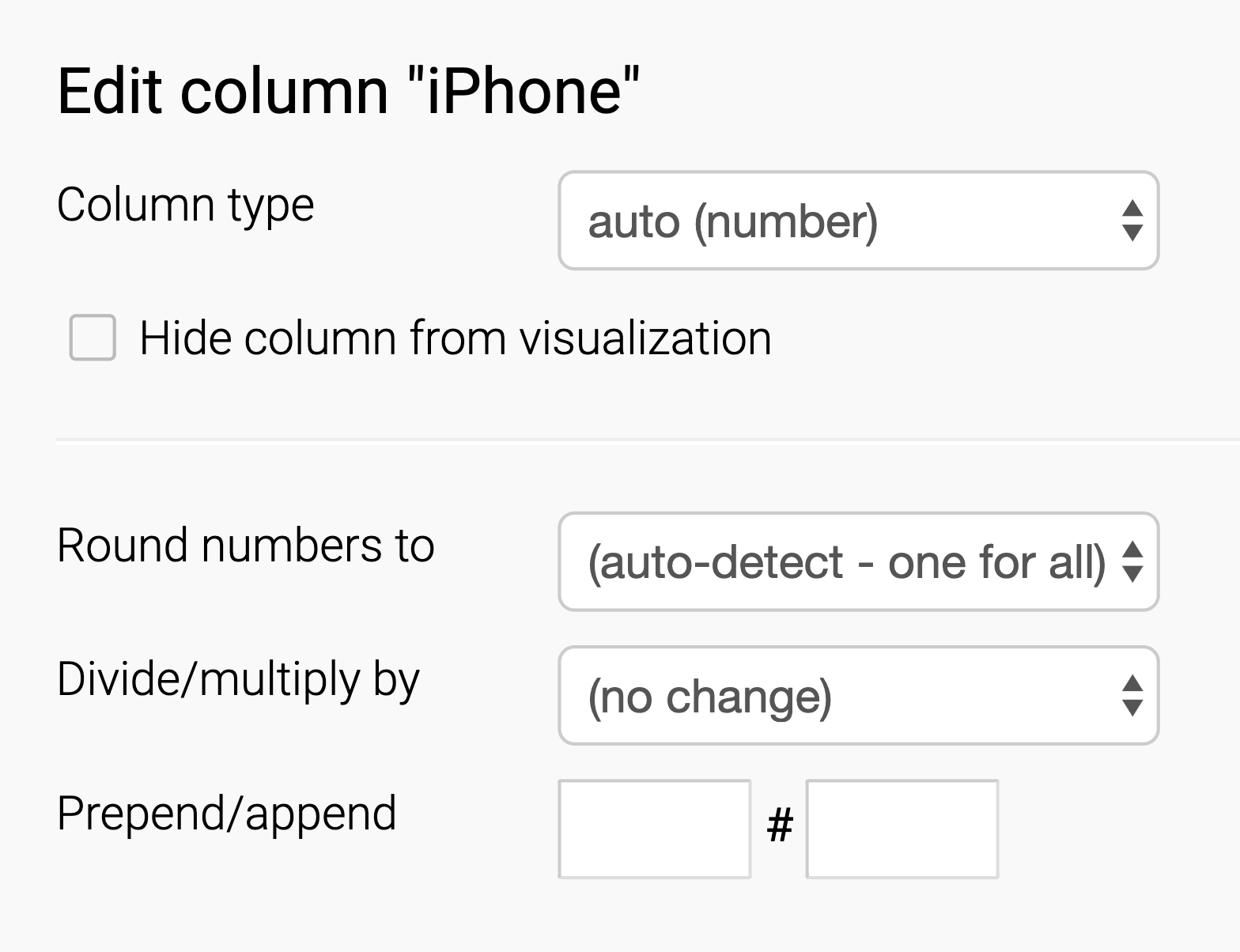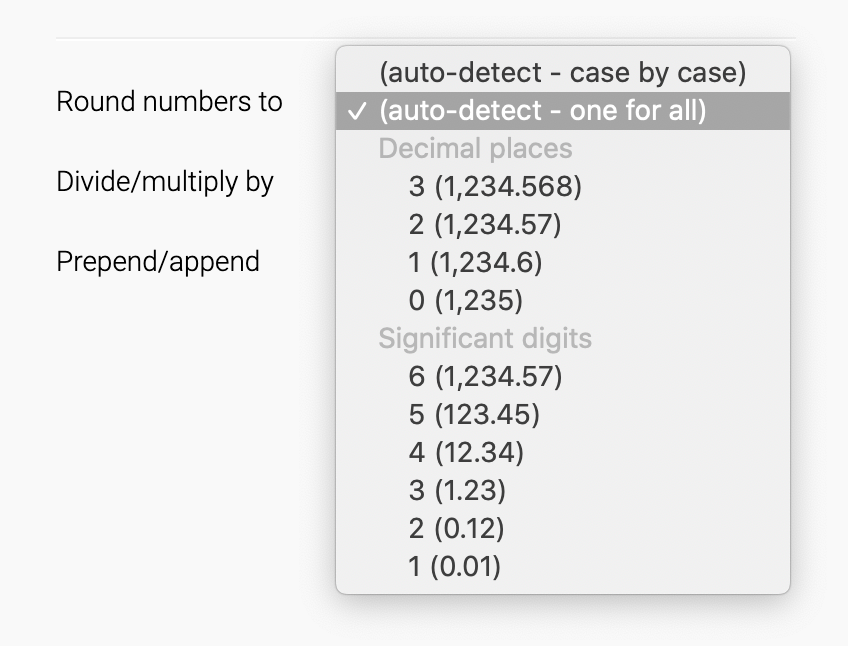# Customize column format: Transform, divide and round numbers

In step 2 of the Datawrapper chart workflow, you will find several helpful, time-saving options to customize the column format and transform the numbers.

To see these options, click on the column header of a number column. The options will then appear on the left.In the example below "iPhone" is the name of the data column.Let's learn when and how to use these options.

⚠️ Transposing the data leads to a re-setting
If you transpose your data table (which means switching the X- and Y-axis), the settings you made so far will vanish. We recommend that you check whether your data structure works well for the chart you're choosing, and then go back to step 2 to perform the options such as rounding, division, prepending, etc. for the final touches.

## Column type

Datawrapper will automatically try to detect the type of data in your column when importing the data. Text is always aligned left, Dates are aligned to the center, numbers are aligned right.  Learn here what data column types exist and how to detect them.

Sometimes, Datwrapper is wrong. If the data type is not correct, this might affect the chart (e.g. you get a tooltip saying "insufficient data"). In this case, you can always go back to step 2 and assign the intended type: Text, Number or Date. (Datawrapper constantly saves your data, so going back and forth does not lead to losing anything.)

## Hide column from visualization

You might have uploaded a range for data columns, but found out later that some are making the whole representation too complex. So, here you can "hide" a certain column. It will not be visualized in the chart then.Note that it is always better to upload just the numbers that are really needed - the option above is something like a quick fix only.

## Round numbers

Here you have a few different choices. Let's assume you have the values 50.05, 20.020, 0.8 and 30.00

• (auto-detect – case by case): This option goes through all values and decides case by case how many decimal places makes sense for your numbers. In our case, the numbers would get rounded to 50.05, 20.02, 0.8 and 30.
• (auto-detect – one for all): This option is the default. It decides for all numbers how many decimal places make sense. In our case, the values would be 50.05, 20.02, 0.8 and 30.00
• Decimal places: Here you can decide on decimal places yourself. E.g. if we use 1, our values would be 50.1, 20.0, 0.8 and 30.0
• Significant digits: With this option, you can choose how many digits in your number you want to display (with the exception of long numbers like 82,293). For example, if we chose 2 digits, our values are 50, 20, 0.80 and 30. If we chose 5 digits, our values are 50.050, 20.020, 0.80000 and 30.000. Note how this is different than selecting decimal places. You can learn more about the concept of significant digits in this video from Khan Academy.## Divide/multiply by

Dividing numbers provides options to correctly switch from very large numbers to shortened, easier to read numbers. Simply divide the original.## Prepend/Append

This feature enables you to add e.g. a Dollar, Euro or % sign (or any other if needed) to describe what the numbers are. You can also add words or phrases, like " candies". The benefit of prepending/appending something in step 2 instead of in step 1 is that your numbers will still get interpreted as numbers, not text.

You can add a space between number and sign if that is the correct annotation.## What about those options below the table in step 2?We prepared extra tutorials on how to use those that you can find below: